## How to convert cm or m to feet and inches in Excel?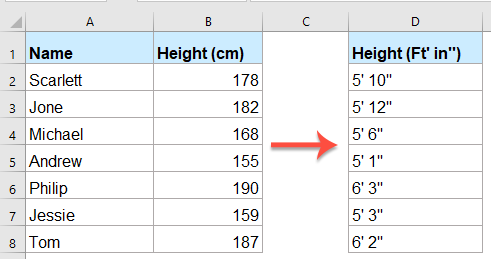If you have a list of centimeter numbers, now, you want to convert these numbers from centimeter to feet and inches as 0’0’’ formatting as below screenshot shown. This article, I will talk about how to convert the centimeters or meters to feet and inches in Excel worksheet.

Convert centimeters to feet and inches with formulas

Convert meters to feet and inches with formulas

#### Convert centimeters to feet and inches with formulas

There is no built-in function for converting the centimeters to feet and inches, but, you can deal with it by using the below formulas:

Please copy and paste the following formula into a blank cell where you want to output the result:

=TRUNC(B2/2.54/12)&"' "&ROUND(MOD(B2/2.54,12),0)&""""

And then, drag the fill handle down to the cells that you want to apply this formula, and you will get the result as below screenshot shown: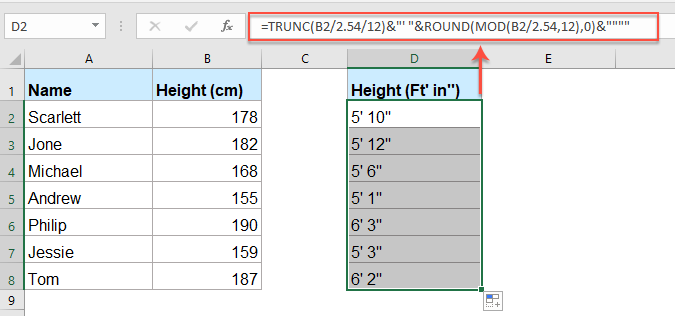Note: If you need to keep two digits after the decimal point for the inches, please apply the below formula:

=INT(CONVERT(B2,"cm","ft")) & "' " & TEXT(12*(CONVERT(B2,"cm","ft")-INT(CONVERT(B2,"cm","ft"))),"0.00") & """"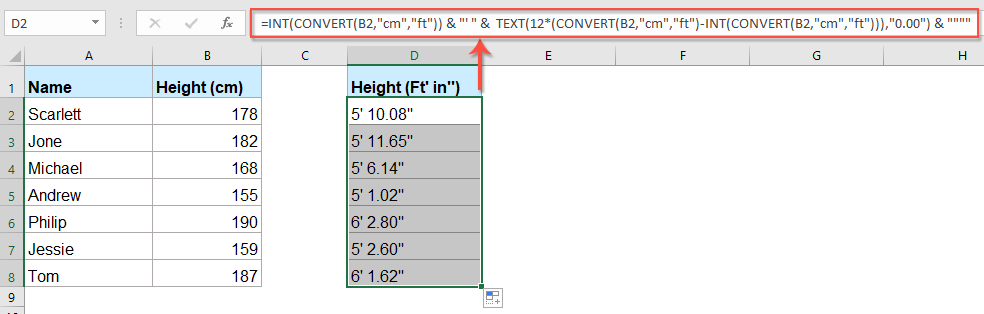#### Convert meters to feet and inches with formulas

If you need to convert meters to feet and inches, you can use the following formulas:

Please copy and paste the below formula into a blank cell:

=TRUNC(B2*100/2.54/12)&"' "&ROUND(MOD(B2*100/2.54,12),0)&""""

And then, drag the fill handle down to the cells to apply this formula, and the meter numbers have been converted to the feet and inches as below screenshot shown: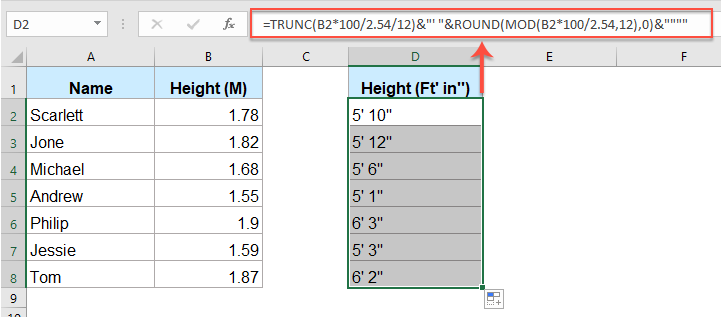Note: If you need to keep two digits after the decimal point for the inches, please apply the below formula:

=INT(CONVERT(B2,"m","ft")) & "' " & TEXT(12*(CONVERT(B2,"m","ft")-INT(CONVERT(B2,"m","ft"))),"0.00") & """"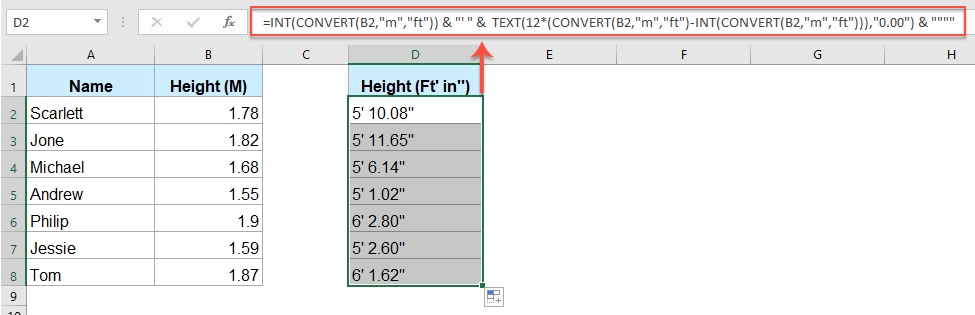## The Best Office Productivity Tools

### Kutools for Excel Solves Most of Your Problems, and Increases Your Productivity by 80%

• Super Formula Bar (easily edit multiple lines of text and formula); Reading Layout (easily read and edit large numbers of cells); Paste to Filtered Range...
• Merge Cells/Rows/Columns and Keeping Data; Split Cells Content; Combine Duplicate Rows and Sum/Average... Prevent Duplicate Cells; Compare Ranges...
• Select Duplicate or Unique Rows; Select Blank Rows (all cells are empty); Super Find and Fuzzy Find in Many Workbooks; Random Select...
• Exact Copy Multiple Cells without changing formula reference; Auto Create References to Multiple Sheets; Insert Bullets, Check Boxes and more...
• Favorite and Quickly Insert Formulas, Ranges, Charts and Pictures; Encrypt Cells with password; Create Mailing List and send emails...
• Extract Text, Add Text, Remove by Position, Remove Space; Create and Print Paging Subtotals; Convert Between Cells Content and Comments...
• Super Filter (save and apply filter schemes to other sheets); Advanced Sort by month/week/day, frequency and more; Special Filter by bold, italic...
• Combine Workbooks and WorkSheets; Merge Tables based on key columns; Split Data into Multiple Sheets; Batch Convert xls, xlsx and PDF...
• Pivot Table Grouping by week number, day of week and more... Show Unlocked, Locked Cells by different colors; Highlight Cells That Have Formula/Name...### Office Tab - brings tabbed interface to Office, and make your work much easier

• Enable tabbed editing and reading in Word, Excel, PowerPoint, Publisher, Access, Visio and Project.
• Open and create multiple documents in new tabs of the same window, rather than in new windows.
• Increases your productivity by 50%, and reduces hundreds of mouse clicks for you every day!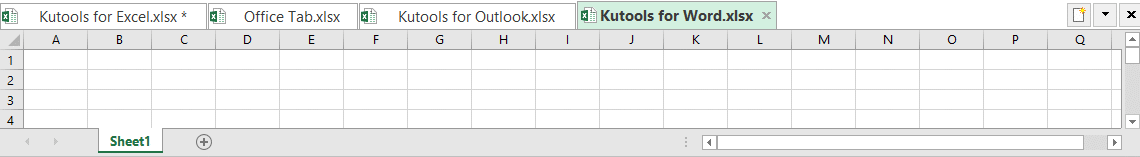No ratings yet. Be the first to rate!
This comment was minimized by the moderator on the site
=INT(CONVERT(B2,"m","ft")) & "' " & TEXT(12*(CONVERT(B2,"m","ft")-INT(CONVERT(B2,"m","ft"))),"0.00") & """"

Regarding to this formula how can you convert it into meters??
This comment was minimized by the moderator on the site
Hello, Jon,
If you want to convert feet and inches format to meters, the following formula may help you:

``=LEFT(A1,SEARCH("'",A1)-1)*0.3048 + (MID(A1,SEARCH("'",A1)+2,SEARCH("""",A1)-SEARCH("'",A1)-2)*0.0254)``

This comment was minimized by the moderator on the site
=INT(CONVERT(B2,"m","ft")) & "' " & TEXT(12*(CONVERT(B2,"m","ft")-INT(CONVERT(B2,"m","ft"))),"0-?/8") & """" to show eights of an inch or ?/4 to show quarters.
This comment was minimized by the moderator on the site
Patrick, this is fantastic - i've created a sheet with a few outputs, i.e. 1/4s, 1/8s, and 1/16s. I'm planning on extending this to include values 1/32, 1/64.

Is there a formula i can use to calculate and display the lowest common denominator value in a separate box?

i.e. 1 ' 3 - 10/16" and 1 ' 3 - 5/8".... i want it to display the 1/8s value in a separate box?
This comment was minimized by the moderator on the site
182 converts to 5' 12". It should be 6' 0" instead.
This comment was minimized by the moderator on the site
182mm equates to 7-1/8". 1.892m or 189.2cm equates to 6' 0".
There are no comments posted here yet